https://doi.org/10.5194/acp-18-17515-2018
https://doi.org/10.5194/acp-18-17515-2018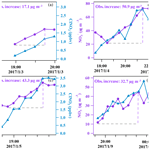# Nitrate formation from heterogeneous uptake of dinitrogen pentoxide during a severe winter haze in southern China

Hui Yun, Weihao Wang, Tao Wang, Men Xia, Chuan Yu, Zhe Wang, Steven C. N. Poon, Dingli Yue, and Yan Zhou
Abstract

Nitrate (${\mathrm{NO}}_{\mathrm{3}}^{-}$) has become a major component of fine particulate matter (PM2.5) during hazy days in China. However, the role of the heterogeneous reactions of dinitrogen pentoxide (N2O5) in nitrate formation is not well constrained. In January 2017, a severe haze event occurred in the Pearl River Delta (PRD) of southern China during which high levels of PM2.5 (∼400µg m−3) and O3 (∼160 ppbv) were observed at a semi-rural site (Heshan) in the western PRD. Nitrate concentrations reached 108 µg m−3 (1 h time resolution), and the contribution of nitrate to PM2.5 was nearly 40 %. Concurrent increases in ${\mathrm{NO}}_{\mathrm{3}}^{-}$ and ClNO2 (with a maximum value of 8.3 ppbv at a 1 min time resolution) were observed in the first several hours after sunset, indicating an intense N2O5 heterogeneous uptake by aerosols. The formation potential of ${\mathrm{NO}}_{\mathrm{3}}^{-}$ via N2O5 heterogeneous reactions was estimated to be between 29.0 and 77.3 µg m−3 in the early hours (2 to 6 h) after sunset based on the measurement data, which could completely explain the measured increase in the ${\mathrm{NO}}_{\mathrm{3}}^{-}$ concentration during the same time period. Daytime production of nitric acid from the gas-phase reaction of OH+NO2 was calculated with a chemical box model built using the Master Chemical Mechanism (MCM v3.3.1) and constrained by the measurement data. The integrated nocturnal nitrate formed via N2O5 chemistry was comparable to or even higher than the nitric acid formed during the day. This study confirms that N2O5 heterogeneous chemistry was a significant source of aerosol nitrate during hazy days in southern China.

Share
Dates
1 Introduction

Severe haze in China has been a major concern of the regulatory and scientific communities in recent years. Nitrate has also been identified as an important component of PM2.5 during hazy days in both summer and winter (e.g., Huang et al., 2014; Li et al., 2018; Pathak et al., 2009; Zhang et al., 2015). Moreover, the proportion of nitrate in PM2.5 has steadily increased over the last decade due to the lagged control of NOx emissions compared to SO2 (Fu et al., 2014; Geng et al., 2017; Qu et al., 2017; Reuter et al., 2014; X. Wang et al., 2016). As a result, the concentrations of nitrate in PM2.5 PM1.0 have been even higher than those of sulfate during some haze events (Ge et al., 2017; Li et al., 2017; Liu et al., 2015; Yang et al., 2017; Yue et al., 2015).

Nitrate is formed from NOx during both the daytime and nighttime. During the day, nitric acid (HNO3) is produced through the gas-phase reaction between OH and NO2 (Reaction R1), while this pathway is insignificant at night due to very low OH concentrations (e.g., Seinfeld and Pandis, 2016). Nitric acid can react with ammonia (NH3) to form ammonium nitrate (NH4NO3), and an equilibrium can be reached for these three compounds between the gas phase and the particle phase (Reactions R2, R3). During nighttime, the heterogeneous uptake of N2O5, which is formed from reactions involving O3, NO2, and NO3, becomes a source of nitrate and also produces gaseous ClNO2 when chloride-containing aerosol is present (Reactions R4–R7; Finlayson-Pitts et al., 1989). This nitrate formation pathway is only important at night due to the fast photolysis of NO3 during the day. Compared to the relatively well-understood formation of aerosol nitrate via the OH+NO2 reaction, the contribution from N2O5 heterogeneous reactions has been poorly quantified due to the limited knowledge of key factors controlling the heterogeneous processes, such as the N2O5 uptake coefficient (${\mathit{\gamma }}_{{\mathrm{N}}_{\mathrm{2}}{\mathrm{O}}_{\mathrm{5}}}$) and ClNO2 yield (${\mathit{\varphi }}_{{\mathrm{ClNO}}_{\mathrm{2}}}$) (Brown and Stutz, 2012; Chang et al., 2011).

$\begin{array}{ll}\text{(R1)}& & \mathrm{OH}+{\mathrm{NO}}_{\mathrm{2}}+\mathrm{M}\to {\mathrm{HNO}}_{\mathrm{3}}+\mathrm{M}\text{(R2)}& & {\mathrm{HNO}}_{\mathrm{3}}\left(g\right)+{\mathrm{NH}}_{\mathrm{3}}\left(g\right)↔{\mathrm{NH}}_{\mathrm{4}}{\mathrm{NO}}_{\mathrm{3}}\left(s\right)\text{(R3)}& & {\mathrm{HNO}}_{\mathrm{3}}\left(g\right)+{\mathrm{NH}}_{\mathrm{3}}\left(g\right)↔{\mathrm{NH}}_{\mathrm{4}}^{+}\left(\mathrm{aq}\right)+{\mathrm{NO}}_{\mathrm{3}}^{-}\left(\mathrm{aq}\right)\text{(R4)}& & {\mathrm{NO}}_{\mathrm{2}}+{\mathrm{O}}_{\mathrm{3}}\to {\mathrm{NO}}_{\mathrm{3}}\text{(R5)}& & {\mathrm{NO}}_{\mathrm{2}}+{\mathrm{NO}}_{\mathrm{3}}+M↔{\mathrm{N}}_{\mathrm{2}}{\mathrm{O}}_{\mathrm{5}}+M\text{(R6)}& & {\mathrm{NO}}_{\mathrm{3}}+\mathrm{VOCs}\to \mathrm{products}& {\mathrm{N}}_{\mathrm{2}}{\mathrm{O}}_{\mathrm{5}}+{\mathrm{H}}_{\mathrm{2}}\mathrm{O}\phantom{\rule{0.25em}{0ex}}\text{or}\phantom{\rule{0.25em}{0ex}}{\mathrm{Cl}}^{-}\left(\mathrm{aq}\right)\\ \text{(R7)}& & \phantom{\rule{0.25em}{0ex}}\phantom{\rule{0.25em}{0ex}}\to \left(\mathrm{2}-\mathit{\varphi }\right){\mathrm{NO}}_{\mathrm{3}}^{-}\left(\mathrm{aq}\right)+\mathit{\varphi }{\mathrm{ClNO}}_{\mathrm{2}}\left(g\right)\end{array}$

Model studies initially treated ${\mathit{\gamma }}_{{\mathrm{N}}_{\mathrm{2}}{\mathrm{O}}_{\mathrm{5}}}$ as a constant (0.03 to 0.1) (Dentener and Crutzen, 1993; Makar et al., 1998; Munger et al., 1998; Schaap et al., 2004; Wen et al., 2015; Xue et al., 2014), and later utilized several parameterization schemes of ${\mathit{\gamma }}_{{\mathrm{N}}_{\mathrm{2}}{\mathrm{O}}_{\mathrm{5}}}$ and ${\mathit{\varphi }}_{{\mathrm{ClNO}}_{\mathrm{2}}}$ based on laboratory investigations of their dependence on aerosol composition and aerosol water content (Anttila et al., 2006; Bertram and Thornton, 2009; Davis et al., 2008; Evans and Jacob, 2005; Riemer et al., 2003, 2009; Roberts et al., 2009). However, recent studies have found a significant discrepancy between the field-derived and the parameterized ${\mathit{\gamma }}_{{\mathrm{N}}_{\mathrm{2}}{\mathrm{O}}_{\mathrm{5}}}$ and ${\mathit{\varphi }}_{{\mathrm{ClNO}}_{\mathrm{2}}}$ (McDuffie et al., 2018; Phillips et al., 2016; Tham et al., 2018; X. Wang et al., 2017; Z. Wang et al., 2017; Zhou et al., 2018). These findings suggest that N2O5 uptake is more complicated than previously thought, and a better understanding of the uptake process is needed to improve the prediction of nitrate and haze.

In addition to the modeling approach, field measurements of trace gases and aerosol composition have been used to infer the contribution of N2O5 heterogeneous chemistry to nitrate formation. Pathak et al. (2009) postulated the importance of N2O5 heterogeneous reactions with respect to the high aerosol nitrate observed in Beijing and Shanghai in summertime by examining the variation of nitrate with the change in relative humidity (RH) and the equilibrium between anions and cations in PM2.5. Pathak et al. (2011) further investigated nitrate formation using a coupled aqueous phase radical mechanism (CAPRAM) and a gas-phase chemistry mechanism (RACM, without ClNO2chemistry). By constraining the uptake coefficient of N2O5 in the range from 0.001 to 0.1, they reproduced the observed enhancement of nitrate and suggested that N2O5 uptake by aerosols contributed to 50 % to 100 % of the nighttime increase in nitrate. A similar method was recently used by Wen et al. (2018) to simulate the summertime nitrate formation in the North China Plain (NCP), which demonstrated the dominant contribution of N2O5 heterogeneous reactions to nighttime nitrate formation. Based on the observed covariation of nitrate and RH, Wang et al. (2009) speculated that N2O5 reactions dominated the nitrate formation on polluted days with high NO2 and O3 concentrations in Shanghai. Neither N2O5 nor ClNO2 was measured during these early observation-based studies. A recent study (H. Wang et al., 2017) inferred ${\mathit{\gamma }}_{{\mathrm{N}}_{\mathrm{2}}{\mathrm{O}}_{\mathrm{5}}}$ from the measured N2O5 on 4 days in urban Beijing and estimated the lower limit of the formation potential of aerosol nitrate assuming a unity ϕClNO2 because ClNO2was not measured. Their result showed a comparable contribution to nitrate formation from the N2O5 heterogeneous chemistry and from the daytime pathway of the OH+NO2 reaction.

In the present study, N2O5, ClNO2, and the related chemical and meteorological parameters were measured at a semi-rural site in the Pearl River Delta of southern China from 2 to 15 January 2017. A severe haze event was observed during the field study with PM2.5 reaching 400 µg m−3 and O3 peaking at 160 ppbv. ClNO2, which is only known to be produced from N2O5 heterogeneous uptake, reached 8.3 ppbv, which is the largest reported value to date and revealed extremely active N2O5 chemistry during the abovementioned episode. The concurrent measurements of N2O5, ClNO2, and aerosol nitrate provide better constraints for elucidating nighttime NO3N2O5 chemistry and aerosol nitrate formation. An overview of the measurement data was first presented. The nighttime processes that led to the formation of nitrate (e.g., the production of NO3 and N2O5, N2O5 uptake coefficient, and ClNO2 yield) were analyzed. The nighttime formation potential of nitrate was estimated based on these data and compared to the measured increase in nitrate. The daytime production of nitric acid via the OH+NO2 reaction was calculated based on a box model using the Master Chemical Mechanism (MCM v3.3.1) and compared to the nighttime formation potential of nitrate.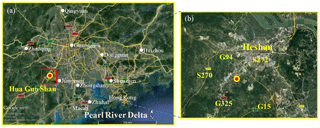Figure 1(a) Google map images of the Pearl River Delta in Guangdong Province and the measurement site (Hua Guo Shan). (b) The topography and major roads (shown by number) adjacent to the measurement site.

2 Methods

## 2.1 Site description

The field observation was conducted at the Guangdong atmospheric supersite, a semi-rural site located at Hua Guo Shan (HGS, 22.728 N, 112.929 E) southwest of the city of Heshan from 2 to 15 January 2017. As shown in Fig. 1, HGS is a hill with a height of 60 m a.s.l. All measurement instruments were located on the fourth floor of a four-story building on the top of the hill. The observation site was located in the western PRD where the economic activity and population density are much lower than the central PRD. There are five main roads near the HGS site, including three national roads (G325, G94, and G15), and two provincial roads (S272 and S270). The hill is covered by subtropical trees and surrounded by similar hills within close proximity; a few residents live at the foot of the hill, and there is some farmland in the area.

## 2.2 Chemical ionization mass spectrometer

N2O5 and ClNO2 were simultaneously observed using a quadrupole chemical ionization mass spectrometer (CIMS, THS Instruments, Atlanta). The same instrument had been used in several previous studies in southern and northern China (Tham et al., 2016; T. Wang et al., 2016; Z. Wang et al., 2017). The reader can refer to these earlier papers for a detailed description of the measurement principle, calibration, and maintenance procedures. Briefly, ambient N2O5 and ClNO2 are converted to ion clusters of I(N2O5) and I(ClNO2) by Iodide ions (I) that are produced by exposing CH3I ∕ N2 (0.3 % vv) to an alpha radioactive source, 210-Po, and are subsequently detected at 235 and 208 m z−1, respectively. Activated carbon packed in a filter was used to determine the instrument background, which were 10.2±2.2 and 8.9±2.0 Hz on average for N2O5 and ClNO2, respectively. In situ offline calibration was carried out every day for N2O5 and every 2 days for ClNO2 by mixing the respective synthetic standard into humidified zero air (with RH controlled at 60 % in the present study). The N2O5 standard was generated by reacting excess NO2 with O3 and determined from the decrease of NO2, and the ClNO2 was synthesized by the uptake of a known concentration of N2O5 on a NaCl slurry (see T. Wang et al., 2016 and Tham et al., 2016 for details). The average sensitivity of N2O5 and ClNO2 was 0.9±0.3 and 0.7±0.2 Hz pptv−1, respectively. The dependence of the sensitivity on the relative humidity was measured during the field study (see Fig. S1) and was used to correct for the RH effect based on the measured ambient RH values. The detection limits of N2O5 and ClNO2 were both 6 pptv (2σ, 1 min-averaged data).

The inlet of the CIMS instrument was set approximately 1.5 m above the roof with 6 m long PFA-Teflon tubing as the sampling line. The total sampling flow was set as 11 standard liters per minute (SLPM). Four SLPM were diverted into the CIMS, the O3 analyzer, and NOx analyzer, and the remaining part was evacuated directly from the system. The total residence time was less than 1 s in the sampling system. Following our previous practice, the inlet tubing and fittings were replaced every afternoon and washed in an ultrasonic bath to reduce the influence of particle deposition on the tubing wall. The loss of N2O5 on the tubing wall was checked on site by injecting N2O5 into the ambient air before and after the tubing replacement; the loss was found to be around 10 % in the“clean” tubing and nearly 40 % by the next afternoon. Because our analysis mainly focused on data from the first few hours of evening, the loss was insignificant and thus was not corrected in our final data. However, this bias could be important in the later period before tube replacement. The uncertainty of the measurement was estimated to be ±25 % for both N2O5 and ClNO2 (T. Wang et al., 2016). The time resolution for the measurement was approximately 10 s, and the derived data were later averaged to a time resolution of 1 min for further analysis.

Table 1Technique, limit of detection, and uncertainty of measuring instruments for trace gases and aerosols.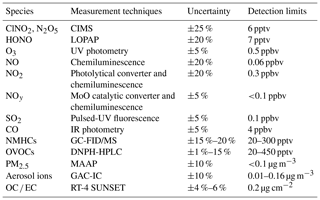## 2.3 Other measurements

Trace gases of CO, SO2, O3, NOx, total reactive nitrogen (NOy), nitrous acid (HONO), C2 to C10 non-methane hydrocarbons (NMHCs), oxygenated hydrocarbons (OVOCs), and aerosol chemical composition and number concentrations were also measured. Table 1 summarizes the principle, detection limit and uncertainty of the measuring instruments.

CO was observed using a gas filter correlation analyzer (Thermo Scientific, Model 48i). SO2 was measured using a pulsed fluorescence analyzer (Thermo Scientific, Model 43i). O3 was determined using a UV photometric analyzer (Thermo Scientific, Model 49i). NO and NO2 were detected with a chemiluminescence instrument (Thermo Scientific, Model 42i) with a photolytic converter to convert NO2 to NO (Xu et al., 2013). NOy was determined using a chemiluminescence analyzer which was equipped with a molybdenum oxide (MoO) catalytic converter (Thermo Scientific, Model 42i-Y). HONO was detected using a long path absorption photometer (QUMA, Model LOPAP-03) (Xu et al., 2015). NMHCs were determined using an online gas chromatograph (GC) coupled with a flame ionization detector (FID) and a mass spectrometer (MS) (Wang et al., 2014). NMHCs were only measured from 2 to 8 January 2017 due to the maintenance of the GCMS after 8 January. OVOCs (e.g., formaldehyde, acetaldehyde, acetone, and methyl ethyl ketone) were sampled using 2,4-dinitrophenylhydrazine (DNPH) cartridges every 3 h and were later analyzed with a high-performance liquid chromatography (HPLC) system (Cui et al., 2016).

Concentrations of PM2.5 were detected with a multi-angle absorption photometer (MAAP; Thermo Scientific, Model 5012). The ionic compositions of PM2.5 were measured with an ion chromatography (GAC-IC) system equipped with a gas and aerosol collector at a time resolution of 30 min (Dong et al., 2012), and the data were also averaged every 1 h to meet the time resolution of other components of PM2.5. Organic carbon (OC) and elemental carbon (EC) were measured using an online OC ∕ EC analyzer (RT-4, SUNSET) with a time resolution of 1 h (Bauer et al., 2009). A scanning mobility particle sizer (SMPS Model 3936L75, TSI) was used to determine the dry-state particle number size distribution, covering the size range from 16.5 to 1000 nm. The ambient (wet) particle number size distributions were estimated based on a size-resolved kappa-Köhler function considering the variation with relative humidity (Hennig et al., 2005; Liu et al., 2014). In the present study, data with a RH greater than 90 % were excluded due to the large uncertainty of the growth factor at very high RH. The aerosol surface area density was then derived using the ambient particle number size distribution (wet) and an assumption of spherical particles with an estimated uncertainty of around 30 % (Tham et al., 2016; Z. Wang et al., 2017).

Meteorological parameters were measured with a portable weather station (WXT520, Vaisala, Finland), including temperature, relative humidity (RH), wind direction, wind speed, and pressure. A pyranometer (CMP22, Kipp & Zonen B.V., Holland) was used to measure the solar radiation, and the data were then utilized to derive the photolysis frequency of NO2 based on the method of Trebs et al. (2009).

## 2.4 Chemical box model

To estimate the daytime formation of nitric acid via the reaction of OH+NO2, an observation-based chemical box model developed with the latest version of the MCM (v3.3.1) (Jenkin et al., 2003, 2015; Saunders et al., 2003) and an updated chlorine (Cl) radical chemistry module (Xue et al., 2015) was utilized to estimate the mixing ratio of OH radicals and the reaction rate of OH+NO2. The integrated production of nitric acid during daytime was then calculated based on the simulation results. The box model was constrained with the observation data every 10 min, including the data relating to N2O5, ClNO2, HONO, O3, NO, NO2, SO2, CO, C2 to C10 NMHCs, OVOCs (formaldehyde, acetaldehyde, acetone, and MEK), temperature, aerosol surface area density, and J(NO2), which were first averaged or interpolated. Average concentrations of NMHC species during the day (07:00 to 17:00) and at night (17:00 to 07:00 of the next day) are shown in Table S1. A function considering the variation of the solar zenith angle (Saunders et al., 2003) was used to calculate the photolysis frequencies of HONO, O3, and other species in clear sky, which were then corrected with the J(NO2) values in the real environment. The J(ClNO2) was treated the same as in Tham et al. (2016). The lifetime of unconstrained species with respect to the physical loss was set as 8 h in a boundary layer with a depth of 1000 m (equivalent to $\mathrm{3.47}×{\mathrm{10}}^{-\mathrm{5}}$ s−1) in order to avoid their accumulation. The model was run from 00:00 on 3 January to 11:00 on 8 January 2017. To stabilize the intermediate species, the simulation for the first 24 h was repeated six times. Sensitivity tests were carried out by reducing the input concentrations by 10 % to check the deviation of the average daytime (07:00–17:00) rate of the OH+NO2 reaction. The simulated rate of the OH+NO2 reaction was most sensitive to HONO, followed by NOx and OVOCs (see Sect. S1 and Fig. S2 in the Supplement).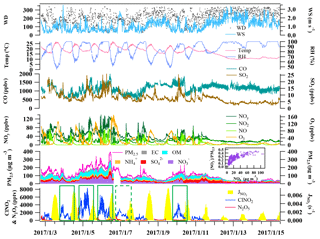Figure 2Time series of N2O5, ClNO2, components of PM2.5, and related trace gases and meteorological parameters from 18:40 on 2 January to 11:00 on 15 January 2017. The inset figure shows the variation of the ratio of nitrate to PM2.5 with an increasing nitrate concentration. The green rectangles in the figure indicate the five days used for detailed analysis.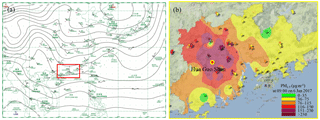Figure 3(a) Surface weather chart at 08:00 LT on 6 January 2017, downloaded from the Hong Kong Observatory website, indicating stagnant conditions. (b) The distribution of PM2.5 concentrations in the PRD region at 09:00 LT on 6 January 2017. This figure was captured from the following website: http://113.108.142.147:20031/GDPublish/publish.aspx (last access: 17 November 2018).

3 Results and discussion

## 3.1 Overview of the observation

Figure 2 shows the time series of N2O5, ClNO2, components of PM2.5, and related trace gases and meteorological parameters from 18:40 on 2 January to 11:00 on 15 January 2017. The average temperature and RH values during the measurement period were 17±4C and 86±14 %, respectively. A severe pollution episode occurred from 3 to 7 January due to stagnant meteorological conditions (Fig. 3a), and the concentrations of most pollutants decreased to very low levels on 9 January and from 12 to 15 January, which corresponded to the change in weather conditions. The most polluted days were 5 and 6 January with the highest PM2.5 value of 400 µg m−3 and the highest O3 concentration of 160 ppbv. The PM2.5 data from the PRD regional air quality monitoring network revealed that the HGS site was within the most polluted area during this haze event (Fig. 3b). This pollution event was characterized by concurrent high levels of PM2.5 and O3 and was in contrast to the winter haze in north China, which experienced high PM2.5 values but low O3 concentrations (e.g., Sun et al., 2016; H. Wang et al., 2018a). The mixing ratios of N2O5 and ClNO2 were up to 3358 and 8324 pptv (1 min time resolution), respectively, indicating active N2O5 heterogeneous chemistry.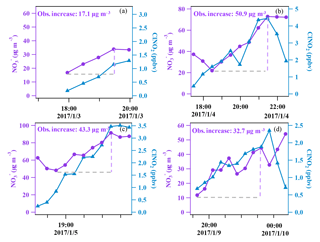Figure 4The covariance of aerosol nitrate and ClNO2 in the early nighttime (at a 30 min time resolution) for four nights.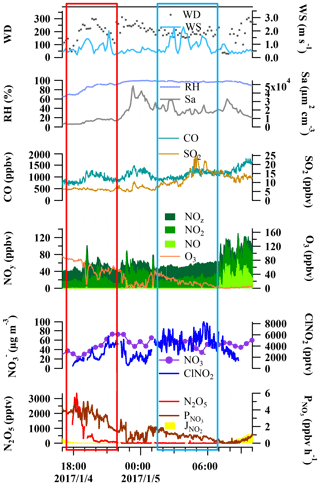Figure 5Variation of N2O5, ClNO2, ${\mathrm{NO}}_{\mathrm{3}}^{-}$, and trace gases and meteorological conditions during the night from 4 to 5 January 2017 as an example from the five selected nights.

Very high concentrations of aerosol nitrate (up to 108 µg m−3; 1 h time resolution) were also observed during the multi-day episode. Nitrate contributed to 24 % of the total PM2.5 mass concentration on average, which was comparable to that of organic matter ($\mathrm{OM}=\mathrm{1.7}\cdot \mathrm{OC}$, 28 %) and much higher than that of sulfate (16 %) and ammonium (11 %). The contribution of nitrate to the PM2.5 increased with an increase in the nitrate concentration, and reached nearly 40 % at its peak, indicating that nitrate was a dominant component of the PM2.5 on the most polluted days. The concentration of ${\mathrm{NO}}_{\mathrm{3}}^{-}$ exhibited a concurrent increase with that of ClNO2 in the early nighttime from 3 to 4, 4 to 5, 5 to 6, and 9 to 10 January (see Fig. 4), suggesting that N2O5 heterogeneous reactions significantly contributed to the formation of nitrate during the night. The measured increases of the ${\mathrm{NO}}_{\mathrm{3}}^{-}$ concentration during these four nights were 17.1, 50.9, 43.3, and 32.7 µg m−3, respectively. A similar increase in ClNO2 was observed from 6 to 7 January, but the composition of the PM2.5 was not available due to instrument maintenance. Apart from chemical reactions, the evolution of the planetary boundary layer (PBL) also affects the concentrations of trace gas and aerosols. The height of PBL generally decreases after sunset due to the fast decrease in the land temperature, which can lead to the accumulation of primary pollutants (and secondary pollutants) at the surface if significant local sources are present. For example, on the night from 4 to 5 January (see Fig. 5), the CO and NOy levels increased between 18:00 and 19:00 LT with the enhancement of ClNO2 and nitrate, indicative of an accumulation of primary emissions; however, after this period of time the primary pollutants decreased for 3 h while ClNO2 and nitrate continued to increase due to the nighttime chemical process.

In the remainder of this paper, we will focus on the detailed analysis of the abovementioned five nights to investigate the role of N2O5 heterogeneous chemistry in nitrate formation.

## 3.2 N2O5 heterogeneous chemistry on the selected nights

### 3.2.1 Production of NO3 and N2O5

The first step in the nighttime nitrate formation via N2O5 chemistry is the production of NO3 and N2O5. To gain insight into the key factors affecting the NO3 ∕ N2O5 chemistry, the variation of N2O5 and the production rate of NO3 were examined in relation to some relevant gases and meteorological parameters on the selected five nights. Figure 5 shows the data for the night from 4 to 5 January as an example. Some common features were identified for all five nights. In general, low wind speed (<2.0 m s−1) at night facilitated the accumulation of air pollutants, and high RH was favorable for N2O5 heterogeneous uptake. In addition, high aerosol surface area density provided interfaces for N2O5 heterogeneous reactions.

In the first couple of hours after sunset (Fig. 5, red rectangle), N2O5 exhibited a peak and then quickly dropped to hundreds of pptv, while nitrate and ClNO2 concurrently increased, which was indicative of the local production and loss of N2O5. NO was below the detection limit during this period. The production rates of NO3 (${P}_{{\mathrm{NO}}_{\mathrm{3}}}={k}_{{\mathrm{NO}}_{\mathrm{2}}+{\mathrm{O}}_{\mathrm{3}}}\left[{\mathrm{NO}}_{\mathrm{2}}\right]\left[{\mathrm{O}}_{\mathrm{3}}\right]\right)$ were the fastest just after sunset and decreased gradually due to reduced O3 levels. There was a period later in the night (22:00 to 01:00) when fresh emissions of NO were observed, and the production of NO3 was suppressed due to the titration of O3 by NO. In the later nighttime, NO was below the detection limit (Fig. 5, blue rectangle). During this period, NO3 and N2O5 were produced at moderate rates, and the very low N2O5 concentrations (below the detection limit) suggested a fast loss of N2O5 probably leading to the local production of ClNO2 and nitrate, which was not revealed in the observed variations of ClNO2 and nitrate. The concentrations of ClNO2 and nitrate during this period fluctuated due to the change in the air masses indicated by the change in SO2 concentrations and wind speeds.

### 3.2.2 The N2O5 uptake coefficient and ClNO2 yield

The N2O5 uptake coefficient and ClNO2 yield, in combination with the reactivity of NO3 with NO and VOCs, determines the loss pathways of NO3 and N2O5. To derive the uptake coefficient of N2O5, a method suggested by McLaren et al. (2010) was applied which treated NO3 and N2O5 as a whole ([NO3]+[N2O5]) without assuming that the chemical system was in a steady state. This approach considers that the change of NO3 and N2O5 concentrations is mainly due to NO3 ∕ N2O5 chemistry; thus, it requires that the air mass has relatively stable chemical conditions and is not subject to fresh NO emissions. It also requires that ClNO2 is produced from the N2O5 chemistry and has an increasing trend to derive the yield of ClNO2. This method is applicable for the early nighttime (Fig. 5, red rectangle; Sect. 3.2.1) for these five nights.

The variation rate of [NO3]+[N2O5] can be calculated by deducting the production rate of [NO3]+[N2O5] with its loss rate as follows:

$\begin{array}{}\text{(1)}& \frac{\mathrm{d}\left(\left[{\mathrm{N}}_{\mathrm{2}}{\mathrm{O}}_{\mathrm{5}}\right]+\left[{\mathrm{NO}}_{\mathrm{3}}\right]\right)}{\mathrm{d}t}={P}_{{\mathrm{NO}}_{\mathrm{3}}}-{L}_{{\mathrm{N}}_{\mathrm{2}}{\mathrm{O}}_{\mathrm{5}}+{\mathrm{NO}}_{\mathrm{3}}}\end{array}$

Table 2Average N2O5 concentration values, N2O5 uptake coefficients, ClNO2 yields, and other related parameters and maximum ClNO2 concentration values in the early nighttime for the five selected nights.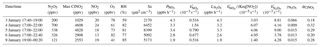The loss of [NO3]+[N2O5] is via the reaction of NO3 with VOCs and N2O5 heterogeneous reactions, which can both be expressed as pseudo-first-order losses:

$\begin{array}{ll}{L}_{{\mathrm{N}}_{\mathrm{2}}{\mathrm{O}}_{\mathrm{5}}+{\mathrm{NO}}_{\mathrm{3}}}& ={L}_{{\mathrm{NO}}_{\mathrm{3}}}+\phantom{\rule{0.125em}{0ex}}{L}_{{\mathrm{N}}_{\mathrm{2}}{\mathrm{O}}_{\mathrm{5}}}\\ \text{(2)}& & ={{k}^{\prime }}_{{\mathrm{NO}}_{\mathrm{3}}}\left[{\mathrm{NO}}_{\mathrm{3}}\right]\phantom{\rule{0.125em}{0ex}}+\phantom{\rule{0.125em}{0ex}}{{k}^{\prime }}_{{\mathrm{N}}_{\mathrm{2}}{\mathrm{O}}_{\mathrm{5}}}\left[{\mathrm{N}}_{\mathrm{2}}{\mathrm{O}}_{\mathrm{5}}\right],\end{array}$

where ${k}_{{\mathrm{NO}}_{\mathrm{3}}}^{\prime }$ and ${k}_{{\mathrm{N}}_{\mathrm{2}}{\mathrm{O}}_{\mathrm{5}}}^{\prime }$ represent the total first-order rate constants for NO3 and N2O5, respectively. The loss rate of N2O5 can then be obtained from Eq. (3):

$\begin{array}{ll}{L}_{{\mathrm{N}}_{\mathrm{2}}{\mathrm{O}}_{\mathrm{5}}}& ={k}_{{\mathrm{N}}_{\mathrm{2}}{\mathrm{O}}_{\mathrm{5}}}^{\prime }\left[{\mathrm{N}}_{\mathrm{2}}{\mathrm{O}}_{\mathrm{5}}\right]=\phantom{\rule{0.125em}{0ex}}{k}_{{\mathrm{NO}}_{\mathrm{2}}+{\mathrm{O}}_{\mathrm{3}}}\left[{\mathrm{NO}}_{\mathrm{2}}\right]\left[{\mathrm{O}}_{\mathrm{3}}\right]\\ \text{(3)}& & -\frac{\mathrm{d}\left[{\mathrm{N}}_{\mathrm{2}}{\mathrm{O}}_{\mathrm{5}}\right]}{\mathrm{d}t}-\frac{\mathrm{d}\left[{\mathrm{NO}}_{\mathrm{3}}\right]}{\mathrm{d}t}-{k}_{{\mathrm{NO}}_{\mathrm{3}}}^{\prime }\left[{\mathrm{NO}}_{\mathrm{3}}\right]\end{array}$

Because NO3 was not measured, it was calculated by assuming an equilibrium of NO2NO3N2O5 as shown in Eq. (4). High levels of NO would break this equilibrium. Thus, the periods with detected NO were excluded. d[NO3] ∕ dt and d[N2O5] ∕ dt were calculated as the rate of change of NO3 and N2O5, respectively, at a time resolution of 10 min. ${k}_{{\mathrm{NO}}_{\mathrm{3}}}^{\prime }$ was derived using the measured concentrations of NMHCs as in Eq. (5) by interpolating the data of the NMHCs to a 10 min time resolution. The NO3 reactivity with VOCs (${k}_{\mathrm{NO}\mathrm{3}}^{\prime }$) in the early nighttime ranged from 0.516 to $\mathrm{1.54}×{\mathrm{10}}^{-\mathrm{3}}$ s−1 (Table 2), which was higher than those derived at Mt. TMS in winter 2013 (0.17 to $\mathrm{1.1}×{\mathrm{10}}^{-\mathrm{3}}$ s−1) (Brown et al., 2016), but lower than those in the North China Plain during the summertime (2 to $\mathrm{57}×{\mathrm{10}}^{-\mathrm{3}}$ s−1) (Tham et al., 2016; H. Wang et al., 2017, 2018b; Z. Wang et al., 2017). NMHCs were not measured from 9 to 10 January 2017. We used the average ${k}_{{\mathrm{NO}}_{\mathrm{3}}}^{\prime }$ in the early nighttime from 3 to 4 January as a replacement because these two periods had similar pollution levels for most pollutants. For the later nighttime (Fig. 5, blue rectangle), low levels of N2O5 and moderate levels of ${P}_{{\mathrm{NO}}_{\mathrm{3}}}$ made Eq. (3) inapplicable even though NO was not detected.

$\begin{array}{}\text{(4)}& & \left[{\mathrm{NO}}_{\mathrm{3}}\right]=\phantom{\rule{0.125em}{0ex}}\frac{\left[{\mathrm{N}}_{\mathrm{2}}{\mathrm{O}}_{\mathrm{5}}\right]}{\left[{\mathrm{NO}}_{\mathrm{2}}\right]\cdot {K}_{\mathrm{eq}}}\text{(5)}& & {{k}^{\prime }}_{{\mathrm{NO}}_{\mathrm{3}}}\phantom{\rule{0.125em}{0ex}}=\phantom{\rule{0.125em}{0ex}}\sum {k}_{i}\left[{\mathrm{VOC}}_{i}\right]\end{array}$

Finally, the uptake coefficient of N2O5 was derived using Eq. (6) for every 10 min and averaged for each selected period. In Eq. (6), ${C}_{{\mathrm{N}}_{\mathrm{2}}{\mathrm{O}}_{\mathrm{5}}}$ is the mean molecular speed of N2O5, and Sa is the aerosol surface area density. The yield of ClNO2 was derived via dividing the integrated production of ClNO2 ([ClNO2]max) by the integrated loss of N2O5 since sunset as Eq. (7).

$\begin{array}{}\text{(6)}& & {{k}^{\prime }}_{{\mathrm{N}}_{\mathrm{2}}{\mathrm{O}}_{\mathrm{5}}}\phantom{\rule{0.125em}{0ex}}=\frac{{L}_{{\mathrm{N}}_{\mathrm{2}}{\mathrm{O}}_{\mathrm{5}}}}{\left[{\mathrm{N}}_{\mathrm{2}}{\mathrm{O}}_{\mathrm{5}}\right]}=\frac{\mathrm{1}}{\mathrm{4}}{C}_{{\mathrm{N}}_{\mathrm{2}}{\mathrm{O}}_{\mathrm{5}}}{S}_{\mathrm{a}}{\mathit{\gamma }}_{{\mathrm{N}}_{\mathrm{2}}{\mathrm{O}}_{\mathrm{5}}}\text{(7)}& & \mathit{\varphi }\phantom{\rule{0.125em}{0ex}}=\phantom{\rule{0.125em}{0ex}}\frac{\left[{\mathrm{ClNO}}_{\mathrm{2}}{\right]}_{\mathrm{max}}}{\int {L}_{{\mathrm{N}}_{\mathrm{2}}{\mathrm{O}}_{\mathrm{5}}}\mathrm{d}t}\end{array}$

The relative importance of the NO3 reactions with VOCs and N2O5 heterogeneous reactions can be examined by comparing the values of the loss coefficient of the NO3 reactions $\left(\frac{{{k}^{\prime }}_{{\mathrm{NO}}_{\mathrm{3}}}}{\left[{\mathrm{NO}}_{\mathrm{2}}\right]\cdot {K}_{\mathrm{eq}}}\right)$ and N2O5 heterogeneous reactions (${k}_{{\mathrm{N}}_{\mathrm{2}}{\mathrm{O}}_{\mathrm{5}}}^{\prime }$) (Tham et al., 2016). Based on the calculations, the values of $\frac{{{k}^{\prime }}_{{\mathrm{NO}}_{\mathrm{3}}}}{\left[{\mathrm{NO}}_{\mathrm{2}}\right]\cdot {K}_{\mathrm{eq}}}$ were $\mathrm{1.40}×{\mathrm{10}}^{-\mathrm{5}}$ to $\mathrm{6.07}×{\mathrm{10}}^{-\mathrm{5}}$ s−1 (see Table 2), while those of ${k}_{{\mathrm{N}}_{\mathrm{2}}{\mathrm{O}}_{\mathrm{5}}}^{\prime }$ were $\mathrm{3.78}×{\mathrm{10}}^{-\mathrm{3}}$ to $\mathrm{9.00}×{\mathrm{10}}^{-\mathrm{3}}$ s−1, which was 2 orders of magnitude higher than $\frac{{{k}^{\prime }}_{{\mathrm{NO}}_{\mathrm{3}}}}{\left[{\mathrm{NO}}_{\mathrm{2}}\right]\cdot {K}_{\mathrm{eq}}}$, suggesting that N2O5 heterogeneous reactions were the dominant loss pathway for both NO3 and N2O5.

Table 3Average values of the N2O5 loss rate and related parameters for selected periods in the later nighttime.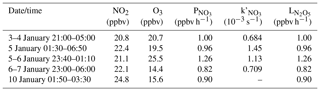The average ${\mathit{\gamma }}_{{\mathrm{N}}_{\mathrm{2}}{\mathrm{O}}_{\mathrm{5}}}$ and ${\mathit{\varphi }}_{{\mathrm{ClNO}}_{\mathrm{2}}}$ derived for the early night in the five cases are listed in Table 2. The data show that the uptake coefficient ranged from 0.009 to 0.066, which was comparable with previous values derived at Mt. Tai Mo Shan (TMS) in Hong Kong (0.004 to 0.022) (Brown et al., 2016) and in the North China Plain (0.006 to 0.102) (Tham et al., 2016, 2018; H. Wang et al., 2017, 2018b; X. Wang et al., 2017; Z. Wang et al., 2017; Zhou et al., 2018). It is interesting to see a much higher ${\mathit{\gamma }}_{{\mathrm{N}}_{\mathrm{2}}{\mathrm{O}}_{\mathrm{5}}}$ value (0.066) on 3 January than those on other four nights (0.009–0.015), which resulted from higher ${P}_{{\mathrm{NO}}_{\mathrm{3}}}$ but much lower Sa and relatively low N2O5 concentrations on 3 January. We examined known factors affecting the loss of NO3 and N2O5 such as the concentrations of NO, NMHCs, and aerosol compositions, but found no obvious difference between 3 January and the other nights. The yield in this study varied from 0.18 to 0.32, which was similar to most studies in China (Tham et al., 2016, 2018; Z. Wang et al., 2017; Yun et al., 2018; Zhou et al., 2018).

The uncertainty of the above ${\mathit{\gamma }}_{{\mathrm{N}}_{\mathrm{2}}{\mathrm{O}}_{\mathrm{5}}}$ was estimated to be ±45 % due to the measurement uncertainty of N2O5 (±25 %), NO2 (±20 %), O3 (±5 %), and Sa (±30 %). The uncertainty of ${\mathit{\varphi }}_{{\mathrm{ClNO}}_{\mathrm{2}}}$ was mainly caused by the uncertainty of NO2 (±20 %), O3 (±5 %), and ClNO2 (±25 %) and was estimated to be ±30 %. The correlation between ${\mathit{\gamma }}_{{\mathrm{N}}_{\mathrm{2}}{\mathrm{O}}_{\mathrm{5}}}$, ${\mathit{\varphi }}_{{\mathrm{ClNO}}_{\mathrm{2}}}$, and the concentrations of aerosol compositions (see Table S2) or RH was investigated, and the results (not shown here) did not indicate any significant dependence of ${\mathit{\gamma }}_{{\mathrm{N}}_{\mathrm{2}}{\mathrm{O}}_{\mathrm{5}}}$ or ${\mathit{\varphi }}_{{\mathrm{ClNO}}_{\mathrm{2}}}$ on these parameters.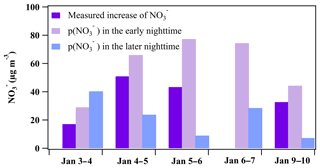Figure 6Comparison between the measured ${\mathrm{NO}}_{\mathrm{3}}^{-}$ increase and the ${\mathrm{NO}}_{\mathrm{3}}^{-}$ formation potential in the early nighttime (periods in Table 2: 3 January 17:40–19:00, 4 January 17:00–22:00, 5 January 17:00–22:00, 6 January 17:00–22:40, and 9 January 19:00–00:20) and in the later nighttime (periods in Table 3: 3–4 January 21:00–05:00, 5 January 01:30–06:50, 5–6 January 23:40–01:10, 6–7 January 23:00–06:00, and 10 January 01:50–03:30).

## 3.3 Nitrate formation potential p(${\mathrm{NO}}_{\mathrm{3}}^{-}$) through N2O5 chemistry

### 3.3.1 Nighttime p(${\mathrm{NO}}_{\mathrm{3}}^{-}$)

The formation potential of ${\mathrm{NO}}_{\mathrm{3}}^{-}$ through N2O5 chemistry is the total amount of ${\mathrm{NO}}_{\mathrm{3}}^{-}$ accumulated from N2O5 heterogeneous loss. It can be calculated by deducting the integrated production of ClNO2 from the integrated loss of N2O5 as follows:

$\begin{array}{ll}p\left({\mathrm{NO}}_{\mathrm{3}}^{-}\right)& =\left(\mathrm{2}-\mathit{\varphi }\right)\int {L}_{{\mathrm{N}}_{\mathrm{2}}{\mathrm{O}}_{\mathrm{5}}}\mathrm{d}t\\ \text{(8)}& & =\phantom{\rule{0.125em}{0ex}}\mathrm{2}\int {L}_{{\mathrm{N}}_{\mathrm{2}}{\mathrm{O}}_{\mathrm{5}}}\mathrm{d}t\phantom{\rule{0.125em}{0ex}}-\phantom{\rule{0.125em}{0ex}}\left[{\mathrm{ClNO}}_{\mathrm{2}}{\right]}_{\mathrm{max}}\end{array}$

In the early nighttime, the average loss rate of N2O5 (${L}_{{\mathrm{N}}_{\mathrm{2}}{\mathrm{O}}_{\mathrm{5}}}$) ranged from 1.9 to 4.3 ppbv h−1 (Table 2), which was close to the average ${P}_{{\mathrm{NO}}_{\mathrm{3}}}$ due to the dominance of the N2O5 heterogeneous reactions in NO3 and N2O5 loss. Based on the derived N2O5 loss rate and the maximum ClNO2 concentration, the formation potential of ${\mathrm{NO}}_{\mathrm{3}}^{-}$ was derived and ranged from 29.0 to 77.3 µg m−3 as shown in Fig. 6. The measured increase of the ${\mathrm{NO}}_{\mathrm{3}}^{-}$ concentration in the early nighttime can be completely explained by the integrated production of ${\mathrm{NO}}_{\mathrm{3}}^{-}$ via the N2O5 heterogeneous reactions during the same period.

In the later nighttime, the method described in Sect. 3.2.2 was not valid for calculating the N2O5 heterogeneous loss rate as mentioned above. We attempted to estimate the formation potential of nitrate by assuming that the N2O5 heterogeneous reactions continued to dominate the loss of NO3+N2O5 in the later nighttime. The ${k}_{{\mathrm{NO}}_{\mathrm{3}}}^{\prime }$ values in the later nighttime were comparable to those in the early nighttime, and the high RH (close to 100 %) in the later nighttime was favorable for N2O5 heterogeneous reactions. We assumed that all NO3 was quickly consumed by the N2O5 heterogeneous reactions, which means that the loss rate of N2O5 approximated to the production rate of NO3 (${L}_{{\mathrm{N}}_{\mathrm{2}}{\mathrm{O}}_{\mathrm{5}}}\approx {P}_{{\mathrm{NO}}_{\mathrm{3}}}$). As listed in Table 3, the N2O5 loss rates ranged from 0.82 to 1.26 ppbv h−1, which were significantly lower than those derived in the early nighttime. The derived N2O5 loss rate here and the yield of ClNO2 in the early nighttime were used to estimate the formation potential of ${\mathrm{NO}}_{\mathrm{3}}^{-}$ in the later nighttime. As shown in Fig. 6, the nitrate produced during these later periods ranged from 7.3 to 40.3 µg m−3, which was lower than values in the early nighttime for four nights, indicating that the nighttime nitrate from N2O5 chemistry was mainly produced in the early nighttime.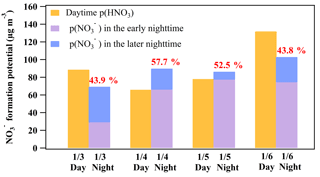Figure 7Comparison between the daytime (07:00 to 17:00 LT; assuming that all gas-phase HNO3 is partitioned into particle phase) and nighttime (17:00 to 07:00 LT of the next day) ${\mathrm{NO}}_{\mathrm{3}}^{-}$ formation potential. The early nighttime of each day represents the periods in Table 2, including 3 January 17:40–19:00, 4 January 17:00–22:00, 5 January 17:00–22:00, 6 January 17:00–22:40, and 9 January 19:00–00:20. The later nighttime of each day represents the periods in Table 3, including 3–4 January 21:00–05:00, 5 January 01:30–06:50, 5–6 January 23:40–01:10, 6–7 January 23:00–06:00, and 10 January 01:50–03:30. The intercomparison of the ${\mathrm{NO}}_{\mathrm{3}}^{-}$ formation potential during the day and night on 9 and 10 January was not conducted due to the lack of NMHC data after 8 January which made the model simulation of OH infeasible during the day on 9 January.

### 3.3.2 Comparison with the daytime production of HNO3

During the daytime, the formation of ${\mathrm{NO}}_{\mathrm{3}}^{-}$ is mainly from the gas–particle partitioning of the gas-phase HNO3 formed via the OH+NO2 reaction. Hence, the daytime formation potential of HNO3 (p(HNO3)) can be treated as the upper limit for the locally produced daytime aerosol nitrate. To calculate the daytime p(HNO3), a box model based on MCM v3.3.1 was used to derive the mixing ratio of OH and the rates of OH+NO2 as described in Sect. 2.4. This model was previously used in our study at Wangdu in northern China (Tham et al., 2016). The calculated mixing ratios of OH at Wangdu with this model compared well with those observed by the laser-induced fluorescence (LIF) technique (Tan et al., 2017). In the present study, the average daytime OH (07:00 to 17:00) mixing ratios were 1.71 to 3.82×106 molec cm−3 from 3 to 7 January, as listed in Table 4, with the maximum values reaching 3.24 to 6.71×106 molec cm−3. The detailed results for OH can be found in Fig. S3.

Table 4Average OH mixing ratio and rate of OH+NO2 during the daytime (07:00 to 17:00 LT) from 3 to 7 January 2017.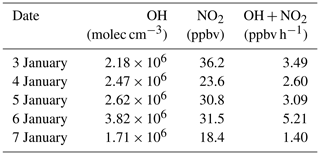The average production rates of HNO3 via the OH+NO2 reaction were 1.40 to 5.21 ppbv h−1 from 3 to 7 January, and the integrated formation potential of HNO3 during the daytime was 35.7 to 131.8 µg m−3, which was comparable to the nighttime p(${\mathrm{NO}}_{\mathrm{3}}^{-}$) that ranged from 69.3 to 102.9 µg m−3 (Fig. 7). The nighttime production of nitrate via the heterogeneous uptake of N2O5 accounted for 43.8 % to 57.7 % of the total nitrate (${\mathrm{NO}}_{\mathrm{3}}^{-}+{\mathrm{HNO}}_{\mathrm{3}}$) produced in a 24 h period at the site. These results underscored the important role of N2O5 heterogeneous chemistry in nitrate formation in this severe winter haze in southern China.

4 Concluding remarks

With the use of concurrent measurements of nitrate, ClNO2, and related pollutants, this study demonstrates the important contribution of N2O5 heterogeneous uptake in nitrate formation. Current chemical transport models have difficulties simulating this nitrate production pathway. Therefore, more research efforts are needed to improve the representations of ${\mathit{\gamma }}_{{\mathrm{N}}_{\mathrm{2}}{\mathrm{O}}_{\mathrm{5}}}$ and ${\mathit{\varphi }}_{{\mathrm{ClNO}}_{\mathrm{2}}}$ for the better prediction of nitrate in the models. The observation-based approach presented here can be applied to investigate nitrate formation in other areas.

Data availability

The data used in this study are available upon request from Tao Wang (cetwang@polyu.edu.hk) and Dingli Yue (dingliyue@163.com).

Supplement

Author contributions

TW designed the research. WW conducted the CIMS measurements, and YZ, DY, HY, MX, CY, and PS performed the measurements of the other parameters used in this study. HY, TW, MX, and WW analyzed the data. HY and TW wrote the paper, and all authors contributed to discussion and commented on the paper.

Competing interests

The authors declare that they have no conflict of interest.

Special issue statement

Acknowledgements

The authors thank Qinyi Li and Xiao Fu for helpful discussions, Yaru Wang and Yiheng Liang for their help with analyzing the OVOC and aerosol composition, and Naiwen Zhang for her help with the HONO measurements. This study was supported by the Research Grants Council of Hong Kong (HK-RGC; C5022-14G and PolyU 153026/14P) and the National Natural Science Foundation (NNSF) of China (91544213). Zhe Wang acknowledges the support of the NNSF of China (41505103) and HK-RGC (25221215).

Edited by: Jingkun Jiang
Reviewed by: three anonymous referees

References

Anttila, T., Kiendler-Scharr, A., Tillmann, R., and Mentel, T. F.: On the reactive uptake of gaseous compounds by organic-coated aqueous aerosols: Theoretical analysis and application to the heterogeneous hydrolysis of N2O5, J. Phys. Chem. A, 110, 10435–10443, 2006.

Bauer, J. J., Yu, X.-Y., Cary, R., Laulainen, N., and Berkowitz, C.: Characterization of the sunset semi-continuous carbon aerosol analyzer, J. Air Waste Manage., 59, 826–833, 2009.

Bertram, T. H. and Thornton, J. A.: Toward a general parameterization of N2O5 reactivity on aqueous particles: the competing effects of particle liquid water, nitrate and chloride, Atmos. Chem. Phys., 9, 8351–8363, https://doi.org/10.5194/acp-9-8351-2009, 2009.

Brown, S. S. and Stutz, J.: Nighttime radical observations and chemistry, Chem. Soc. Rev., 41, 6405–6447, 2012.

Brown, S. S., Dubé, W. P., Tham, Y. J., Zha, Q., Xue, L., Poon, S., Wang, Z., Blake, D. R., Tsui, W., and Parrish, D. D.: Nighttime Chemistry at a High Altitude Site Above Hong Kong, J. Geophys. Res.-Atmos., 121, 2457–2475, https://doi.org/10.1002/2015JD024566, 2016.

Chang, W. L., Bhave, P. V., Brown, S. S., Riemer, N., Stutz, J., and Dabdub, D.: Heterogeneous Atmospheric Chemistry, Ambient Measurements, and Model Calculations of N2O5: A Review, Aerosol Sci. Tech., 45, 665–695, https://doi.org/10.1080/02786826.2010.551672, 2011.

Cui, L., Zhang, Z., Huang, Y., Lee, S. C., Blake, D. R., Ho, K. F., Wang, B., Gao, Y., Wang, X. M., and Louie, P. K. K.: Measuring OVOCs and VOCs by PTR-MS in an urban roadside microenvironment of Hong Kong: relative humidity and temperature dependence, and field intercomparisons, Atmos. Meas. Tech., 9, 5763–5779, https://doi.org/10.5194/amt-9-5763-2016, 2016.

Davis, J. M., Bhave, P. V., and Foley, K. M.: Parameterization of N2O5 reaction probabilities on the surface of particles containing ammonium, sulfate, and nitrate, Atmos. Chem. Phys., 8, 5295–5311, https://doi.org/10.5194/acp-8-5295-2008, 2008.

Dentener, F. J. and Crutzen, P. J.: Reaction of N2O5 on tropospheric aerosols: Impact on the global distributions of NOx, O3, and OH, J. Geophys. Res.-Atmos., 98, 7149–7163, 1993.

Dong, H.-B., Zeng, L.-M., Hu, M., Wu, Y.-S., Zhang, Y.-H., Slanina, J., Zheng, M., Wang, Z.-F., and Jansen, R.: Technical Note: The application of an improved gas and aerosol collector for ambient air pollutants in China, Atmos. Chem. Phys., 12, 10519–10533, https://doi.org/10.5194/acp-12-10519-2012, 2012.

Evans, M. J. and Jacob, D. J.: Impact of new laboratory studies of N2O5 hydrolysis on global model budgets of tropospheric nitrogen oxides, ozone, and OH, Geophys. Res. Lett., 32, L09813, https://doi.org/10.1029/2005GL022469, 2005.

Finlayson-Pitts, B. J., Ezell, M. J., and Pitts, J. N.: Formation of chemically active chlorine compounds by reactions of atmospheric NaCl particles with gaseous N2O5 and ClONO2, Nature, 337, 241–244, 1989.

Fu, X., Wang, X., Guo, H., Cheung, K., Ding, X., Zhao, X., He, Q., Gao, B., Zhang, Z., and Liu, T.: Trends of ambient fine particles and major chemical components in the Pearl River Delta region: observation at a regional background site in fall and winter, Sci. Total Environ., 497, 274–281, 2014.

Ge, X., He, Y., Sun, Y., Xu, J., Wang, J., Shen, Y., and Chen, M.: Characteristics and formation mechanisms of fine particulate nitrate in typical urban areas in China, Atmosphere, 8, 62, https://doi.org/10.3390/atmos8030062, 2017.

Geng, G., Zhang, Q., Tong, D., Li, M., Zheng, Y., Wang, S., and He, K.: Chemical composition of ambient PM2.5 over China and relationship to precursor emissions during 2005–2012, Atmos. Chem. Phys., 17, 9187–9203, https://doi.org/10.5194/acp-17-9187-2017, 2017.

Hennig, T., Massling, A., Brechtel, F., and Wiedensohler, A.: A tandem DMA for highly temperature-stabilized hygroscopic particle growth measurements between 90 % and 98 % relative humidity, J. Aerosol Sci., 36, 1210–1223, 2005.

Huang, R. J., Zhang, Y. L., Bozzetti, C., Ho, K. F., Cao, J. J., Han, Y. M., Daellenbach, K. R., Slowik, J. G., Platt, S. M., Canonaco, F., Zotter, P., Wolf, R., Pieber, S. M., Bruns, E. A., Crippa, M., Ciarelli, G., Piazzalunga, A., Schwikowski, M., Abbaszade, G., Schnelle-Kreis, J., Zimmermann, R., An, Z. S., Szidat, S., Baltensperger, U., El Haddad, I., and Prevot, A. S. H.: High secondary aerosol contribution to particulate pollution during haze events in China, Nature, 514, 218–222, https://doi.org/10.1038/Nature13774, 2014.

Jenkin, M. E., Saunders, S. M., Wagner, V., and Pilling, M. J.: Protocol for the development of the Master Chemical Mechanism, MCM v3 (Part B): tropospheric degradation of aromatic volatile organic compounds, Atmos. Chem. Phys., 3, 181–193, https://doi.org/10.5194/acp-3-181-2003, 2003.

Jenkin, M. E., Young, J. C., and Rickard, A. R.: The MCM v3.3.1 degradation scheme for isoprene, Atmos. Chem. Phys., 15, 11433–11459, https://doi.org/10.5194/acp-15-11433-2015, 2015.

Li, H., Zhang, Q., Zheng, B., Chen, C., Wu, N., Guo, H., Zhang, Y., Zheng, Y., Li, X., and He, K.: Nitrate-driven urban haze pollution during summertime over the North China Plain, Atmos. Chem. Phys., 18, 5293–5306, https://doi.org/10.5194/acp-18-5293-2018, 2018.

Liu, H. J., Zhao, C. S., Nekat, B., Ma, N., Wiedensohler, A., van Pinxteren, D., Spindler, G., Müller, K., and Herrmann, H.: Aerosol hygroscopicity derived from size-segregated chemical composition and its parameterization in the North China Plain, Atmos. Chem. Phys., 14, 2525–2539, https://doi.org/10.5194/acp-14-2525-2014, 2014.

Li, W., Liu, X., Zhang, Y., Sun, K., Wu, Y., Xue, R., Zeng, L., Qu, Y., and An, J.: Characteristics and formation mechanism of regional haze episodes in the Pearl River Delta of China, J. Environ. Sci., 63, 236–249, 2017.

Liu, X., Sun, K., Qu, Y., Hu, M., Sun, Y., Zhang, F., and Zhang, Y.: Secondary formation of sulfate and nitrate during a haze episode in megacity Beijing, China, Aerosol Air Qual. Res., 15, 2246–2257, 2015.

Makar, P., Wiebe, H., Staebler, R., Li, S., and Anlauf, K.: Measurement and modeling of particle nitrate formation, J. Geophys. Res.-Atmos., 103, 13095–13110, 1998.

McDuffie, E. E., Fibiger, D. L., Dubé, W. P., Lopez-Hilfiker, F., Lee, B. H., Thornton, J. A., Shah, V., Jaeglé, L., Guo, H., and Weber, R. J.: Heterogeneous N2O5 uptake during winter: Aircraft measurements during the 2015 WINTER campaign and critical evaluation of current parameterizations, J. Geophys. Res.-Atmos., 123, 4345–4372, https://doi.org/10.1002/2018JD028336, 2018.

McLaren, R., Wojtal, P., Majonis, D., McCourt, J., Halla, J. D., and Brook, J.: NO3 radical measurements in a polluted marine environment: links to ozone formation, Atmos. Chem. Phys., 10, 4187–4206, https://doi.org/10.5194/acp-10-4187-2010, 2010.

Munger, J. W., Fan, S. M., Bakwin, P. S., Goulden, M. L., Goldstein, A., Colman, A. S., and Wofsy, S. C.: Regional budgets for nitrogen oxides from continental sources: Variations of rates for oxidation and deposition with season and distance from source regions, J. Geophys. Res.-Atmos., 103, 8355–8368, 1998.

Pathak, R. K., Wu, W. S., and Wang, T.: Summertime PM2.5 ionic species in four major cities of China: nitrate formation in an ammonia-deficient atmosphere, Atmos. Chem. Phys., 9, 1711–1722, https://doi.org/10.5194/acp-9-1711-2009, 2009.

Pathak, R. K., Wang, T., and Wu, W. S.: Nighttime enhancement of PM2.5 nitrate in ammonia-poor atmospheric conditions in Beijing and Shanghai: Plausible contributions of heterogeneous hydrolysis of N2O5 and HNO3 partitioning, Atmos. Environ., 45, 1183–1191, https://doi.org/10.1016/j.atmosenv.2010.09.003, 2011.

Phillips, G. J., Thieser, J., Tang, M., Sobanski, N., Schuster, G., Fachinger, J., Drewnick, F., Borrmann, S., Bingemer, H., Lelieveld, J., and Crowley, J. N.: Estimating N2O5 uptake coefficients using ambient measurements of NO3, N2O5, ClNO2 and particle-phase nitrate, Atmos. Chem. Phys., 16, 13231–13249, https://doi.org/10.5194/acp-16-13231-2016, 2016.

Qu, Z., Henze, D. K., Capps, S. L., Wang, Y., Xu, X., Wang, J., and Keller, M.: Monthly top-down NOx emissions for China (2005–2012): A hybrid inversion method and trend analysis, J. Geophys. Res.-Atmos., 122, 4600–4625, 2017.

Reuter, M., Buchwitz, M., Hilboll, A., Richter, A., Schneising, O., Hilker, M., Heymann, J., Bovensmann, H., and Burrows, J.: Decreasing emissions of NOx relative to CO2 in East Asia inferred from satellite observations, Nat. Geosci., 7, 792–795, https://doi.org/10.1038/NGEO2257, 2014.

Riemer, N., Vogel, H., Vogel, B., Schell, B., Ackermann, I., Kessler, C., and Hass, H.: Impact of the heterogeneous hydrolysis of N2O5 on chemistry and nitrate aerosol formation in the lower troposphere under photosmog conditions, J. Geophys. Res., 108, 4144, https://doi.org/10.1029/2002JD002436, 2003.

Riemer, N., Vogel, H., Vogel, B., Anttila, T., Kiendler, Scharr, A., and Mentel, T.: Relative importance of organic coatings for the heterogeneous hydrolysis of N2O5 during summer in Europe, J. Geophys. Res., 114, D17307, https://doi.org/10.1029/2008JD011369, 2009.

Roberts, J. M., Osthoff, H. D., Brown, S. S., Ravishankara, A., Coffman, D., Quinn, P., and Bates, T.: Laboratory studies of products of N2O5 uptake on Cl-containing substrates, Geophys. Res. Lett., 36, L20808, https://doi.org/10.1029/2009GL040448, 2009.

Saunders, S. M., Jenkin, M. E., Derwent, R. G., and Pilling, M. J.: Protocol for the development of the Master Chemical Mechanism, MCM v3 (Part A): tropospheric degradation of non-aromatic volatile organic compounds, Atmos. Chem. Phys., 3, 161–180, https://doi.org/10.5194/acp-3-161-2003, 2003.

Schaap, M., van Loon, M., ten Brink, H. M., Dentener, F. J., and Builtjes, P. J. H.: Secondary inorganic aerosol simulations for Europe with special attention to nitrate, Atmos. Chem. Phys., 4, 857–874, https://doi.org/10.5194/acp-4-857-2004, 2004.

Seinfeld, J. H. and Pandis, S. N.: Atmospheric chemistry and physics: from air pollution to climate change, John Wiley & Sons, New York, 2016.

Sun, Y., Chen, C., Zhang, Y., Xu, W., Zhou, L., Cheng, X., Zheng, H., Ji, D., Li, J., and Tang, X.: Rapid formation and evolution of an extreme haze episode in Northern China during winter 2015, Sci. Rep., 6, 27151, https://doi.org/10.1038/srep27151, 2016.

Tan, Z., Fuchs, H., Lu, K., Hofzumahaus, A., Bohn, B., Broch, S., Dong, H., Gomm, S., Häseler, R., He, L., Holland, F., Li, X., Liu, Y., Lu, S., Rohrer, F., Shao, M., Wang, B., Wang, M., Wu, Y., Zeng, L., Zhang, Y., Wahner, A., and Zhang, Y.: Radical chemistry at a rural site (Wangdu) in the North China Plain: observation and model calculations of OH, HO2 and RO2 radicals, Atmos. Chem. Phys., 17, 663–690, https://doi.org/10.5194/acp-17-663-2017, 2017.

Tham, Y. J., Wang, Z., Li, Q., Yun, H., Wang, W., Wang, X., Xue, L., Lu, K., Ma, N., Bohn, B., Li, X., Kecorius, S., Größ, J., Shao, M., Wiedensohler, A., Zhang, Y., and Wang, T.: Significant concentrations of nitryl chloride sustained in the morning: investigations of the causes and impacts on ozone production in a polluted region of northern China, Atmos. Chem. Phys., 16, 14959–14977, https://doi.org/10.5194/acp-16-14959-2016, 2016.

Tham, Y. J., Wang, Z., Li, Q., Wang, W., Wang, X., Lu, K., Ma, N., Yan, C., Kecorius, S., Wiedensohler, A., Zhang, Y., and Wang, T.: Heterogeneous N2O5 uptake coefficient and production yield of ClNO2 in polluted northern China: roles of aerosol water content and chemical composition, Atmos. Chem. Phys., 18, 13155–13171, https://doi.org/10.5194/acp-18-13155-2018, 2018.

Trebs, I., Bohn, B., Ammann, C., Rummel, U., Blumthaler, M., Königstedt, R., Meixner, F. X., Fan, S., and Andreae, M. O.: Relationship between the NO2 photolysis frequency and the solar global irradiance, Atmos. Meas. Tech., 2, 725–739, https://doi.org/10.5194/amt-2-725-2009, 2009.

Wang, H., Lu, K., Chen, X., Zhu, Q., Chen, Q., Guo, S., Jiang, M., Li, X., Shang, D., Tan, Z., Wu, Y., Wu, Z., Zou, Q., Zheng, Y., Zeng, L., Zhu, T., Hu, M., and Zhang, Y.: High N2O5 Concentrations Observed in Urban Beijing: Implications of a Large Nitrate Formation Pathway, Environ. Sci. Tech. Lett., 4, 416–420, https://doi.org/10.1021/acs.estlett.7b00341, 2017.

Wang, H., Lu, K., Chen, X., Zhu, Q., Wu, Z., Wu, Y., and Sun, K.: Fast particulate nitrate formation via N2O5 uptake aloft in winter in Beijing, Atmos. Chem. Phys., 18, 10483–10495, https://doi.org/10.5194/acp-18-10483-2018, 2018a.

Wang, H., Lu, K., Guo, S., Wu, Z., Shang, D., Tan, Z., Wang, Y., Le Breton, M., Lou, S., Tang, M., Wu, Y., Zhu, W., Zheng, J., Zeng, L., Hallquist, M., Hu, M., and Zhang, Y.: Efficient N2O5 uptake and NO3 oxidation in the outflow of urban Beijing, Atmos. Chem. Phys., 18, 9705–9721, https://doi.org/10.5194/acp-18-9705-2018, 2018b.

Wang, M., Zeng, L., Lu, S., Shao, M., Liu, X., Yu, X., Chen, W., Yuan, B., Zhang, Q., Hu, M., and Zhang, Z.: Development and validation of a cryogen-free automatic gas chromatograph system (GC-MS/FID) for online measurements of volatile organic compounds, Anal. Methods, 6, 9424–9434, https://doi.org/10.1039/C4AY01855A, 2014.

Wang, T., Tham, Y. J., Xue, L., Li, Q., Zha, Q., Wang, Z., Poon, S. C., Dubé, W. P., Blake, D. R., and Louie, P. K.: Observations of nitryl chloride and modeling its source and effect on ozone in the planetary boundary layer of southern China, J. Geophys. Res.-Atmos., 121, 2476–2489, https://doi.org/10.1002/2015JD024556, 2016.

Wang, X., Zhang, Y., Chen, H., Yang, X., Chen, J., and Geng, F.: Particulate nitrate formation in a highly polluted urban area: a case study by single-particle mass spectrometry in Shanghai, Environ. Sci. Technol., 43, 3061–3066, 2009.

Wang, X., Chen, W., Chen, D., Wu, Z., and Fan, Q.: Long-term trends of fine particulate matter and chemical composition in the Pearl River Delta Economic Zone (PRDEZ), China, Front. Environ. Sci. Eng., 10, 53–62, 2016.

Wang, X., Wang, H., Xue, L., Wang, T., Wang, L., Gu, R., Wang, W., Tham, Y. J., Wang, Z., Yang, L., Chen, J., and Wang, W.: Observations of N2O5 and ClNO2 at a polluted urban surface site in North China: High N2O5 uptake coefficients and low ClNO2 product yields, Atmos. Environ., 156, 125–134, 2017.

Wang, Z., Wang, W., Tham, Y. J., Li, Q., Wang, H., Wen, L., Wang, X., and Wang, T.: Fast heterogeneous N2O5 uptake and ClNO2 production in power plant and industrial plumes observed in the nocturnal residual layer over the North China Plain, Atmos. Chem. Phys., 17, 12361–12378, https://doi.org/10.5194/acp-17-12361-2017, 2017.

Wen, L., Chen, J., Yang, L., Wang, X., Xu, C., Sui, X., Yao, L., Zhu, Y., Zhang, J., and Zhu, T.: Enhanced formation of fine particulate nitrate at a rural site on the North China Plain in summer: The important roles of ammonia and ozone, Atmos. Environ., 101, 294–302, 2015.

Wen, L., Xue, L., Wang, X., Xu, C., Chen, T., Yang, L., Wang, T., Zhang, Q., and Wang, W.: Summertime fine particulate nitrate pollution in the North China Plain: increasing trends, formation mechanisms and implications for control policy, Atmos. Chem. Phys., 18, 11261–11275, https://doi.org/10.5194/acp-18-11261-2018, 2018.

Xu, Z., Wang, T., Xue, L. K., Louie, P. K. K., Luk, C. W. Y., Gao, J., Wang, S. L., Chai, F. H., and Wang, W. X.: Evaluating the uncertainties of thermal catalytic conversion in measuring atmospheric nitrogen dioxide at four differently polluted sites in China, Atmos. Environ., 76, 221–226, 2013.

Xu, Z., Wang, T., Wu, J., Xue, L., Chan, J., Zha, Q., Zhou, S., Louie, P. K. K., and Luk, C. W. Y.: Nitrous acid (HONO) in a polluted subtropical atmosphere: Seasonal variability, direct vehicle emissions and heterogeneous production at ground surface, Atmos. Environ., 106, 100–109, 2015.

Xue, J., Yuan, Z., Lau, A. K., and Yu, J. Z.: Insights into factors affecting nitrate in PM2.5 in a polluted high NOx environment through hourly observations and size distribution measurements, J. Geophys. Res.-Atmos., 119, 4888–4902, 2014.

Xue, L. K., Saunders, S. M., Wang, T., Gao, R., Wang, X. F., Zhang, Q. Z., and Wang, W. X.: Development of a chlorine chemistry module for the Master Chemical Mechanism, Geosci. Model Dev., 8, 3151–3162, https://doi.org/10.5194/gmd-8-3151-2015, 2015.

Yang, T., Sun, Y., Zhang, W., Wang, Z., Liu, X., Fu, P., and Wang, X.: Evolutionary processes and sources of high-nitrate haze episodes over Beijing, Spring, J. Environ. Sci., 54, 142–151, 2017.

Yue, D., Zhong, L., Zhang, T., Shen, J., Zhou, Y., Zeng, L., Dong, H., and Ye, S.: Pollution properties of water-soluble secondary inorganic ions in atmospheric PM2.5 in the Pearl River Delta region, Aerosol Air Qual. Res., 15, 1737–1747, 2015.

Yun, H., Wang, T., Wang, W., Tham, Y. J., Li, Q., Wang, Z., and Poon, S. C. N.: Nighttime NOx loss and ClNO2 formation in the residual layer of a polluted region: Insights from field measurements and an iterative box model, Sci. Total Environ., 622–623, 727–734, 2018.

Zhang, R., Wang, G., Guo, S., Zamora, M. L., Ying, Q., Lin, Y., Wang, W., Hu, M., and Wang, Y.: Formation of Urban Fine Particulate Matter, Chem. Rev., 115, 3803–3855, https://doi.org/10.1021/acs.chemrev.5b00067, 2015.

Zhou, W., Zhao, J., Ouyang, B., Mehra, A., Xu, W., Wang, Y., Bannan, T. J., Worrall, S. D., Priestley, M., Bacak, A., Chen, Q., Xie, C., Wang, Q., Wang, J., Du, W., Zhang, Y., Ge, X., Ye, P., Lee, J. D., Fu, P., Wang, Z., Worsnop, D., Jones, R., Percival, C. J., Coe, H., and Sun, Y.: Production of N2O5 and ClNO2 in summer in urban Beijing, China, Atmos. Chem. Phys., 18, 11581–11597, https://doi.org/10.5194/acp-18-11581-2018, 2018.